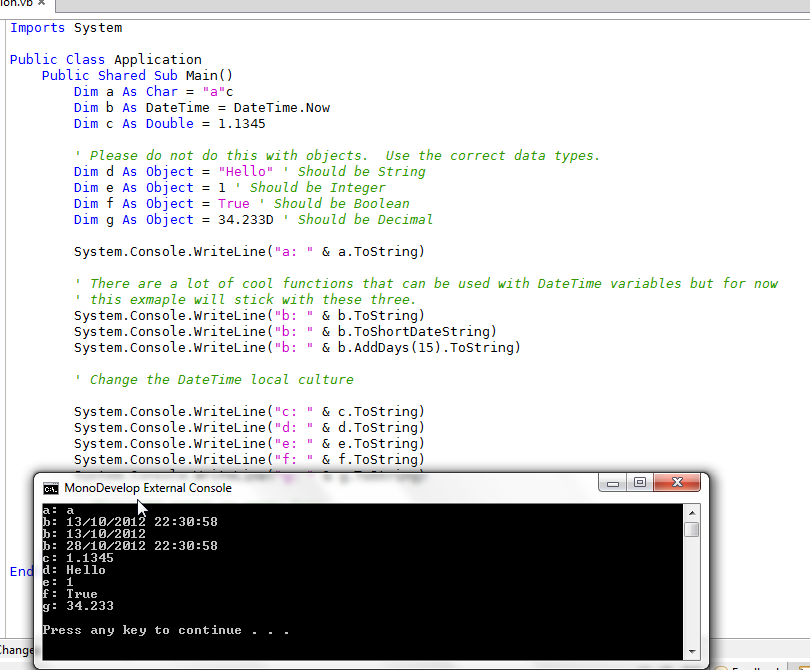The variable types discussed in this lesson are not really any more advanced the the last lesson. I just wanted to split them into their own lesson.

To see the example code that goes along with lesson go to https://github.com/majorsilence/VB-Notes/blob/master/VbBook1/AdvancedVariables/Application.vb

The four types that will be covered are:

• Char
• DateTime
• Double
• Object

# Chars

Chars are variables that can hold one character and only one character. It can be any character available but only one character at a time.

To declare a char you do this.

``````Dim c As Char
``````

This example creates a new variable of type Char named c. It does not assign any value to c. To assign a value to c you can do it like this.

``````c="A"c
``````

Now the value of c is A.

``````c="~"c
``````

Now the value of c is ~.

As is written above any character can be held in a char variable but only one character at a time. Like any other variable type you can print the variable to the console using like this.

``````System.Console.WriteLine(c)
``````

# DateTime

DateTime variables can hold a date and time value. If you just want a date you can also use Date instead of DateTime.

To declare a DateTime you do this.

``````Dim t As DateTime
``````

This example creates a new variable of type DateTime named t. It does not assign any value to t. To assign a value to t you can do it like this.

``````t=DateTime.Now
``````

This assigns the current date and time to the variable t.

If you want to assign a specific date such as 01 may 2012, do it like this.

``````t=New DateTime(2012, 5, 1)
``````

Now the date of t would now be 01 May 2012 with a time of 00:00:00.

If you want to print the date to the console you can use several of its functions to print in different formats.

``````System.Console.WriteLine(t.ToString)
System.Console.WriteLine(t.ToShortDateString)
``````

There are several other functions that can be looked up and used but generally I find these are the two that I use most often.

# Doubles

Doubles are variables that hold numeric values with decimal places. They are similar to the decimal variable type but are less accurate and accumulate rounding errors when calculations are performed. See the lesson Doubles are Bad - Financial Calculations for more information on this.

To declare a double you do this.

``````Dim d As Double
``````

This example creates a new variable of type Double named d. It does not assign any value to d. To assign a value to d you can do it like this.

``````d=5.555567
``````

Now the value of d is 5.555567.

``````d=2.1
``````

Now the value of d is 2.1.

Like any other variable type you can print the variable to the console using like this.

``````System.Console.WriteLine(d)
``````

#Objects Objects are a base type that all other objects are derived from. This means that any other variable no matter the type can be assigned to an object.

To declare an object you do this.

``````Dim o As Object
``````

This example creates a new variable of type Object named o. It does not assign any value to o. To assign a value to o you can do it like this.

``````o="A"c
``````

Now the value of o is A. The type is Char stored in the object. If we assign an integer.

``````o=120
``````

Now the value of o is 120 and the type of the stored value is an Integer

We can do the same by assigning strings, decimals, doubles, or any other type or object into an object of type Object.

If we print the object when assigned an integer it will print the integer. If we print when it is assigned char it will print the char and so on with the other variable types.

``````System.Console.WriteLine(o)
``````

Generally I suggest avoiding the Object type as it defeats type checking that a compiler does and in my experience causes a lot of run time errors. The runtime errors are caused when code attempts to do an operation on the object that is not supported by the stored variable type. If we declare the type we want to use in code the compiler can do all the checks that are needed when the program is complied.

# Screenshot

This screenshot shows the example code linked above running.